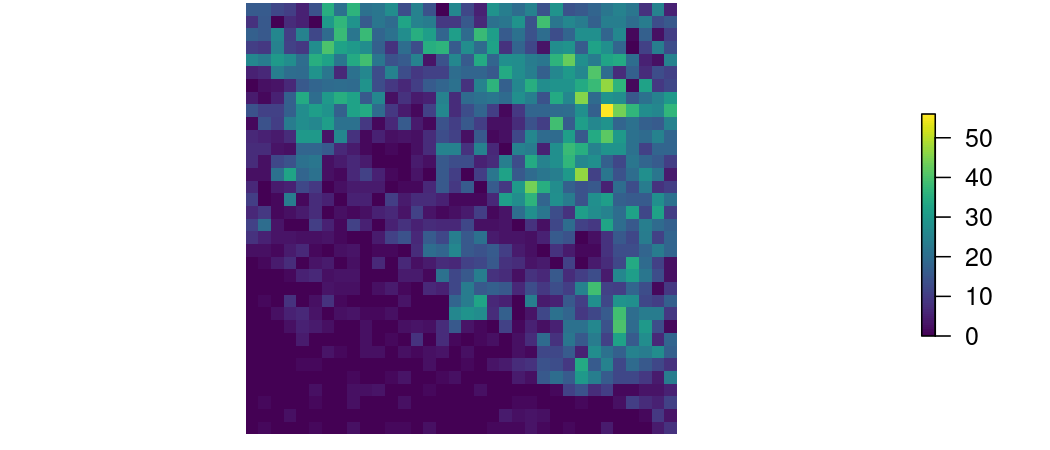# An Eastern Grey Kangaroo population simulation example

#### 2022-10-05

Below is an example using the Eastern Grey Kangaroo (EGK) - a large marsupial native to Australia. The required packages can be loaded using the following:

library(steps)
library(raster)
library(viridisLite)
library(future)

First we setup a stage-based transition matrix, also known as a Lefkovitch matrix. The first matrix represents transition probabilities for each of the life stages in our kangaroo model. In this matrix, fecundities - or numbers of offspring per surviving and contributing stage - are in the first row. These are always represented as positive numbers. Survival probabilities are shown in the remaining rows and are expressed as positive numbers between zero and one. The second matrix describes the uncertainty around these transition probabilities (as standard deviations) and is used to simulate environmental stochasticity - zeros indicating no stochasticity, i.e. there is no uncertainty about environmental fluctuations in our system. Note, there are three life-stages and the matrices are symmetrical in the number of columns and rows (three columns, three rows). Also, names are added to the columns and rows to identify the different life-stages of the kangaroo. These example transition matrix and stochasticity matrix objects are available in the steps package (type “egk_mat” or “egk_mat_stoch” at the R prompt to access).

egk_mat <- matrix(c(0.00,0.55,0.85,
0.50,0.20,0.00,
0.00,0.55,0.85),
nrow = 3,
ncol = 3,
byrow = TRUE)

egk_mat_stoch <- matrix(c(0.00,0.05,0.05,
0.05,0.05,0.00,
0.00,0.01,0.01),
nrow = 3,
ncol = 3,
byrow = TRUE)
colnames(egk_mat_stoch) <- rownames(egk_mat_stoch) <- c('juvenile','subadult','adult')

Next, we read in spatial inputs to be used for the simulations - steps operates primarily on raster data. A 17.5km x 18km spatial grid with a pixel resolution of 500m x 500m is used as the landscape for the metapopulation of kangaroos. This is considered a grid-based metapopulation structure because each cell represents a population that kangaroos can move to and from - dependent upon its unique attributes.

A habitat suitability layer describes the relative likelihood of the environment to support organisms (the kangaroo in this example) in each cell and should contain values between 0 (inhabitable) and 1 (habitable). If the original values are not in this range, they should be rescaled accordingly. This example habitat suitability layer is available in the steps package (type “egk_hab” at the R prompt to access), however, any raster can be used in its place.

egk_hab
## class      : RasterLayer
## dimensions : 35, 36, 1260  (nrow, ncol, ncell)
## resolution : 500, 500  (x, y)
## extent     : 319000, 337000, 5817000, 5834500  (xmin, xmax, ymin, ymax)
## crs        : +proj=utm +zone=55 +south +ellps=GRS80 +towgs84=0,0,0,0,0,0,0 +units=m +no_defs
## source     : memory
## names      : EGK_habitat_500
## values     : 0.003082677, 0.9678264  (min, max)
par(mar=c(0,0,0,0), oma=c(0,0,0,0))
plot(egk_hab, box = FALSE, axes = FALSE, col = viridis(100), main = "Habitat Suitability")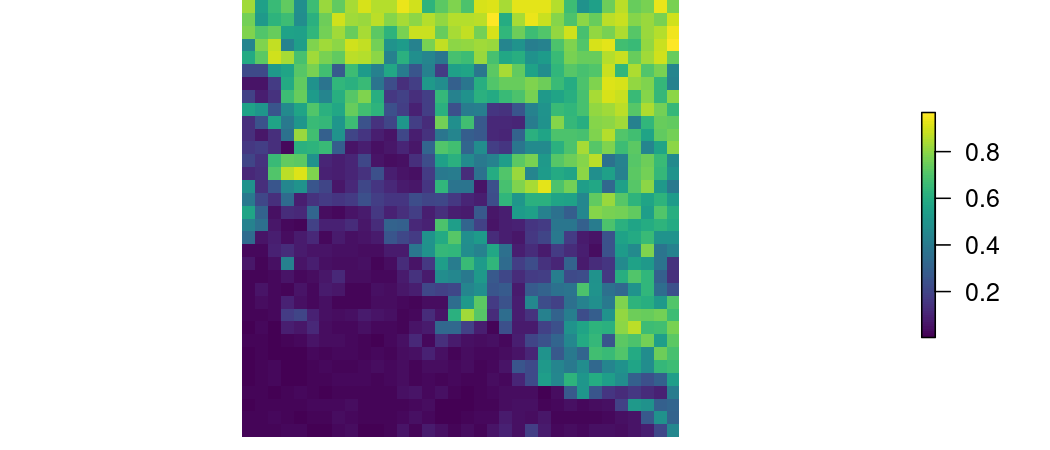The carrying capacity layer describes the total number of organisms (here, kangaroos) that may occur in each cell and contains either zeros or positive integer values. This example carrying capacity raster is available in the steps package (type “egk_k” at the R prompt to access), however, any raster can be used in its place. The example carrying capacity is directly based on the example habitat suitability, which is why the rasters look similar, but this won’t always be the case.

egk_k
## class      : RasterLayer
## dimensions : 35, 36, 1260  (nrow, ncol, ncell)
## resolution : 500, 500  (x, y)
## extent     : 319000, 337000, 5817000, 5834500  (xmin, xmax, ymin, ymax)
## crs        : +proj=utm +zone=55 +south +ellps=GRS80 +towgs84=0,0,0,0,0,0,0 +units=m +no_defs
## source     : memory
## names      : layer
## values     : 1, 73  (min, max)
par(mar=c(0,0,0,0), oma=c(0,0,0,0))
plot(egk_k, box = FALSE, axes = FALSE, col = viridis(100))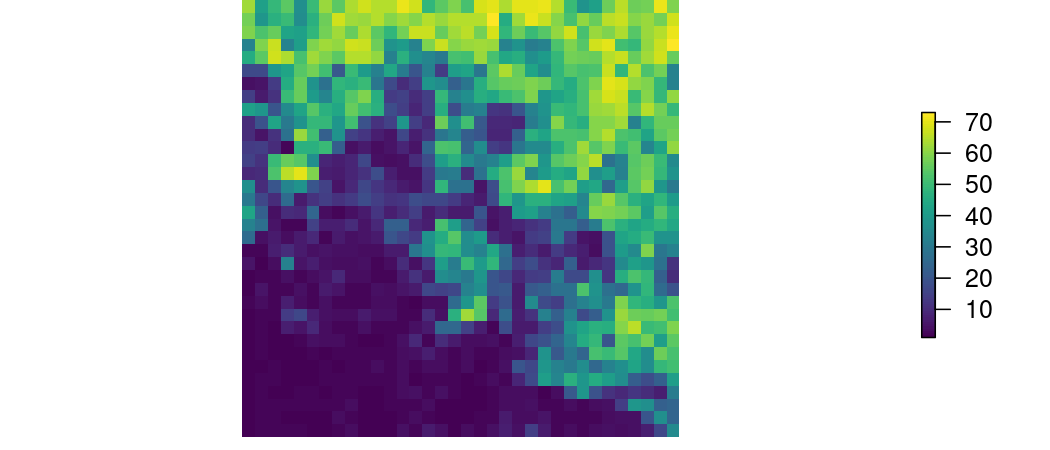Populations are represented as a stack of rasters that describes the total number of individuals that occur in each cell for each life-stage at the beginning of the simulations (initial populations). In the kangaroo example data, there are three life-stages and thus three individual raster layers in the stack. The values are either zeros or positive integers. This example population abundance stack is available in the steps package (type “egk_pop” at the R prompt to access), however, any raster stack can be used in its place.

egk_pop
## class      : RasterStack
## dimensions : 35, 36, 1260, 3  (nrow, ncol, ncell, nlayers)
## resolution : 500, 500  (x, y)
## extent     : 319000, 337000, 5817000, 5834500  (xmin, xmax, ymin, ymax)
## crs        : +proj=utm +zone=55 +south +ellps=GRS80 +towgs84=0,0,0,0,0,0,0 +units=m +no_defs
## min values :        1,        1,     1
## max values :       20,       20,    20
par(mar=c(0,0,0,0), oma=c(0,0,0,0))
spplot(egk_pop, col.regions = viridis(100))
## Warning in wkt(obj): CRS object has no comment

## Warning in wkt(obj): CRS object has no comment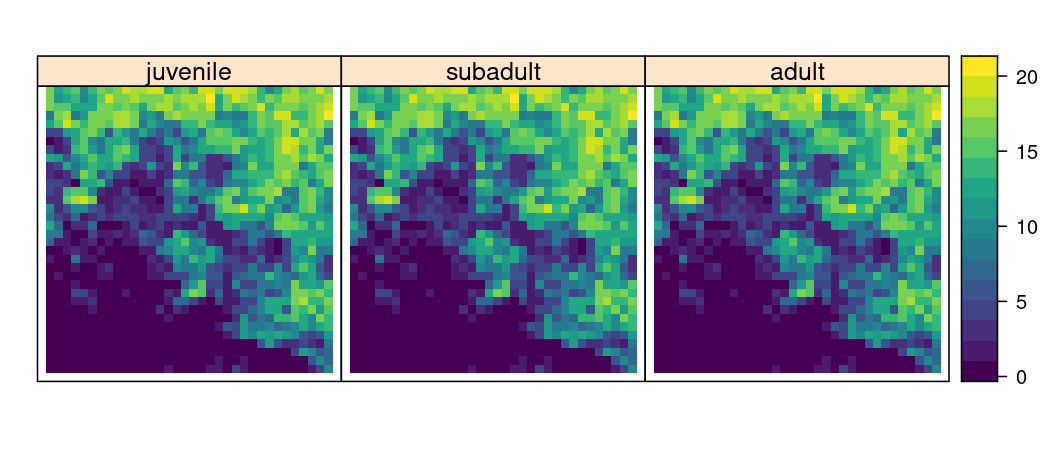For this example, we have used equal numbers of kangaroos in each cell for each life-stage. This, of course, will not always be the case. For example, a user may derive initial population abundances by using stable state age distributions multiplied by the carrying_capacity of the landscape, and then drawn from a multinomial distribution to return whole integers:

library(foreach)
stable_states <- abs( eigen(egk_mat)$vectors[,1] / sum(eigen(egk_mat)$vectors[,1]))
popN <- stack(replicate(ncol(egk_mat), egk_k)) * stable_states
idx <- which(!is.na(getValues(popN[])))
pop <- stack(
foreach(i = 1:nlayers(popN)) %do% {
max_pop <- ceiling(cellStats(popN[[i]], max, na.rm = T))
pop_values <- popN[[i]][idx]
popN[[i]][idx] <- rbinom(prob = (pop_values/max_pop),
size = max_pop,
n = length(pop_values))
popN[[i]]
})
names(pop) <- colnames(egk_mat)
pop
## class      : RasterStack
## dimensions : 35, 36, 1260, 3  (nrow, ncol, ncell, nlayers)
## resolution : 500, 500  (x, y)
## extent     : 319000, 337000, 5817000, 5834500  (xmin, xmax, ymin, ymax)
## crs        : +proj=utm +zone=55 +south +ellps=GRS80 +towgs84=0,0,0,0,0,0,0 +units=m +no_defs
## min values :        0,        0,     0
## max values :       29,       16,    29

All three of these spatial components (habitat suitability, carrying capacity, and initial stage abundances) are combined to define a “landscape” object, however, only the population abundance stack (egk_pop) is required to complete a simulation. Note, all input rasters in the simulations need to have the same projection, extent, resolution, and positions of null (NA) values. The landscape object is modified at each timestep in a single simulation based on population and habitat dynamics (described in subsequent sections below).

egk_landscape <- landscape(population = egk_pop,
suitability = NULL,
carrying_capacity = NULL)

Above are the only landscape data input requirements for a simple population simulation, however, we also need to specify population dynamics. Dynamic functions are used to modify populations or habitats in a landscape at each timestep in a simulation. They can be selected as ‘off-the-shelf’ functions - i.e. those included in the steps package - or custom defined functions created by the user. Four types of population dynamics can be specified: change in population sizes resulting from transition matrices, population dispersal around the landscape, modifications to the population size and configuration, and density dependence. In its most basic form, only population change will be applied to the landscape. As shown in the following code, we use the growth() function, which uses the probabilities in the transition matrix to change the population, and leave all of the other parameters at their default values (NULL). This is analogous to specifying a simple life-stage population growth model.

egk_pop_dynamics <- population_dynamics(change = growth(transition_matrix = egk_mat),
dispersal = NULL,
modification = NULL,
density_dependence = NULL)

Now that we have constructed the landscape object and defined a dynamic object, we can run a single simulation (i.e replicates = 1 which is the default). We simulate changes to the kangaroo population over twenty timesteps. Runtime will depend on the complexity of the landscape object and the configuration of the dynamic object(s). The “verbose = FALSE” suppresses information printed to the console during a simulation; if omitted or set to TRUE (default) the simulation progress bars are shown.

egk_results <- simulation(landscape = egk_landscape,
population_dynamics = egk_pop_dynamics,
habitat_dynamics = NULL,
timesteps = 20,
replicates = 1,
verbose = FALSE)

Once a simulation has been run, we can plot spatially-explicit and temporally-explicit information. For the simulation run above, we can view the kangaroo population trajectories of each life-stage:

plot_pop_trend(egk_results)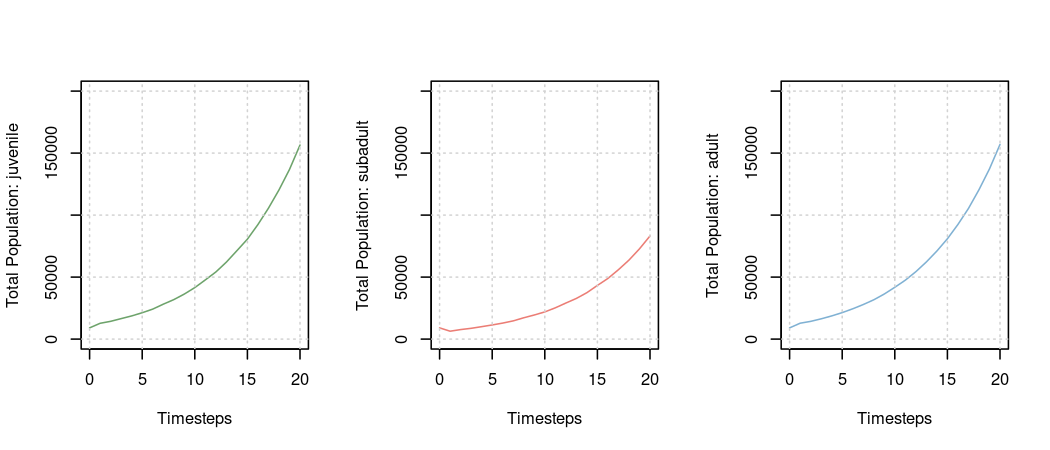Or view the total kangaroo population trajectory (by setting the stage parameter to 0 - type ?plot_pop_trend for more information):

plot_pop_trend(egk_results, stage = 0)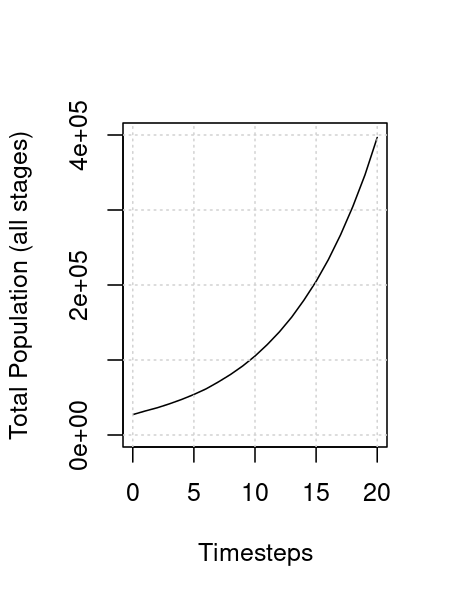Or view the kangaroo population trajectory for a single life-stage:

plot_pop_trend(egk_results, stage = 2)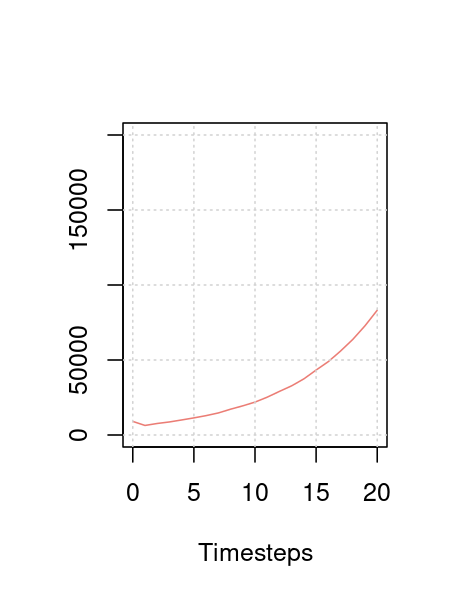We can also view the population distribution over the landscape for a single life-stage (only timesteps one, ten, and twenty shown) - type ?plot_pop_spatial for more information:

plot_pop_spatial(egk_results, stage = 2, timesteps = c(1, 10, 20))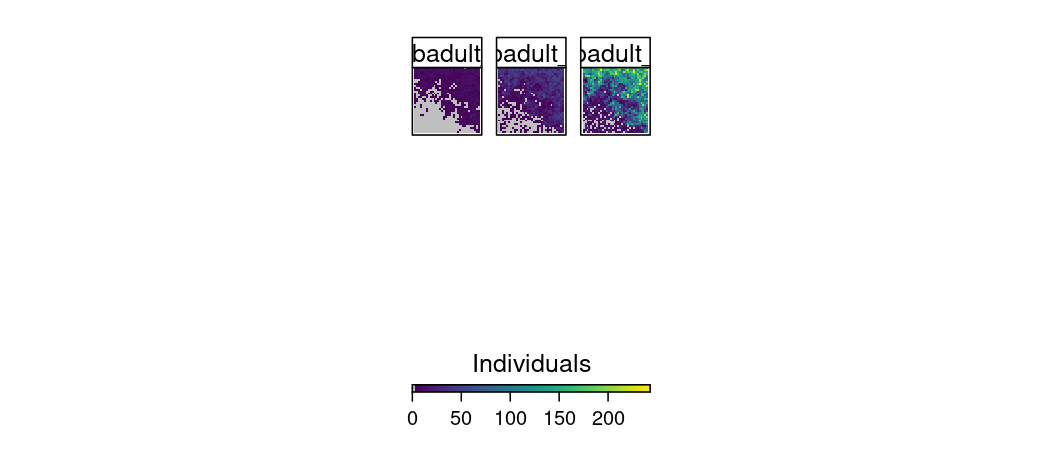To view the changes in population distribution over time, all of the trends and rasters may also be plotted as animations. Here is code to animate the the population trends and rasters of subadults for all timesteps:

plot(egk_results)

We can also generate multiple replicates of a simulation. For the kangaroo, we specify three replicates of a twenty timestep simulation. The replicates are run sequentially by default, however, to improve computation time, we have included the ability to run all three replicates in parallel - each on a different processor. Type ?plan for more information on how to operate in parallel. Note plan() is only required to be called once at the beginning of a script.

plan(multisession, workers = 3) # This is how we specify to simulate
# replicates on separate processors in parallel

egk_results <- simulation(landscape = egk_landscape,
population_dynamics = egk_pop_dynamics,
habitat_dynamics = NULL,
timesteps = 20,
replicates = 3,
verbose = FALSE)

Note, if several replicates of a simulation are run, we must explicitly specify which one to plot for spatial visualisation (default is the first). The components of the landscape object are stored for each timestep (if they are originally specified) so it is possible to also view the population throughout a single simulation. Note, only timesteps one, ten, and twenty are shown - this is controlled by specifying the “timesteps” parameter in the plot call:

plot_pop_spatial(egk_results, replicate = 1, timesteps = c(1, 10, 20))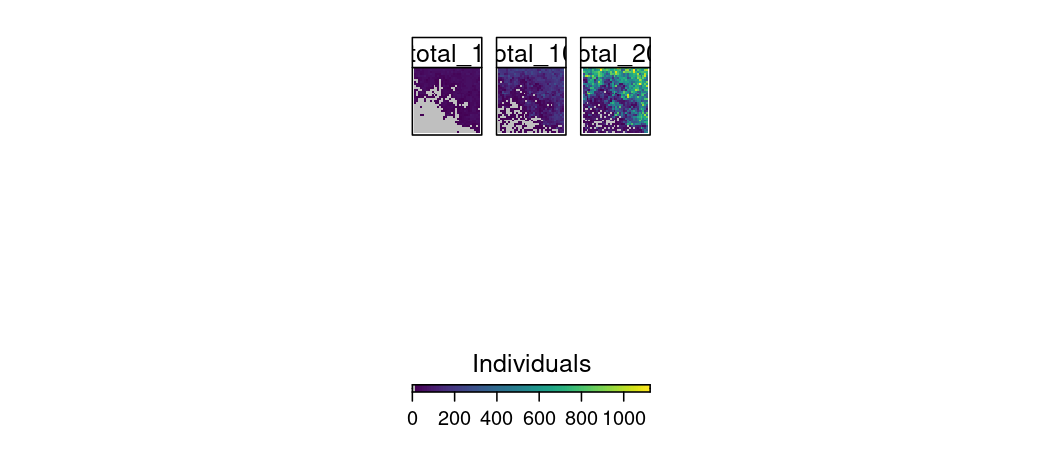Up to this point, the projected population changes in a multiple replicate simulation will all be similar to the population projection in the single replicate simulation. This is because we have not added any stochastic dynamics to the landscape. However, demographic stochasticity IS on by default because it’s incorporated into the transition probabilities that the growth function uses - this behaviour can be disabled by setting ‘demo_stochasticity = “none”’ in the simulation parameters. Let’s try adding some globally-acting (applies to all cells in the landscape) environmental stochasticity to the growth function:

egk_pop_dynamics <- population_dynamics(change = growth(transition_matrix = egk_mat,
global_stochasticity = egk_mat_stoch),
dispersal = NULL,
modification = NULL,
density_dependence = NULL)

egk_results <- simulation(landscape = egk_landscape,
population_dynamics = egk_pop_dynamics,
habitat_dynamics = NULL,
demo_stochasticity = "none",
timesteps = 20,
replicates = 3,
verbose = FALSE)

plot_pop_trend(egk_results)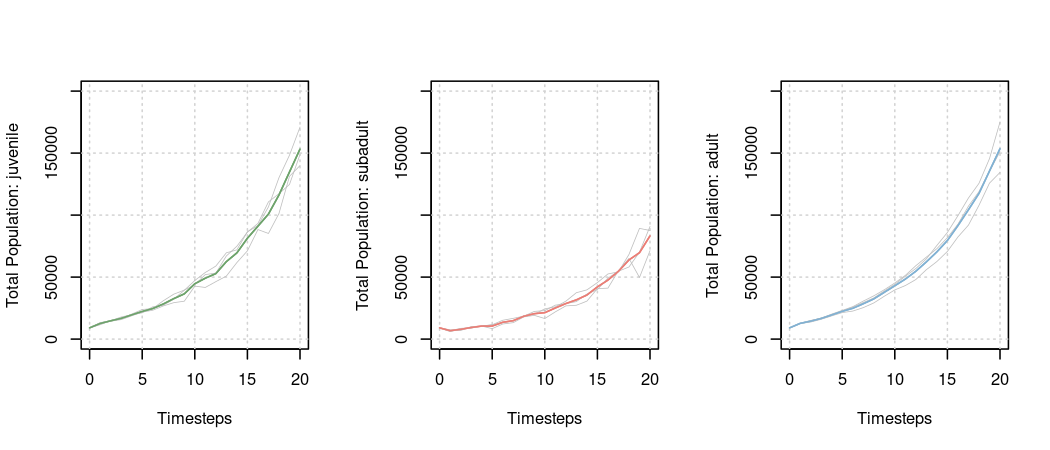We can also specify how survival and fecundity values are influenced by spatial layers in the landscape object (e.g. habitat suitability, carrying capacity, etc.). We modify the survival and fecundity values in the transition matrix at each time step according to a spatial layer by specifying a transition function within the growth function. This is done by multiplying values in the spatial layers by the survival and fecundity values in the transition matrix. This produces new survival and fecundity values for each grid cell in the landscape. Note, if either the survival or fecundity layer are set to NULL (default), the respective values in the transition matrix will remain unchanged. A user may add any spatial layer(s) to the landscape object to modify the transition matrices; here, we specify the habitat suitability layer in the landscape for the survival only:

plan(sequential)

egk_landscape <- landscape(population = egk_pop,
suitability = egk_hab,
carrying_capacity = NULL)

egk_pop_dynamics <- population_dynamics(
change = growth(transition_matrix = egk_mat,
global_stochasticity = egk_mat_stoch,
transition_function = modified_transition(survival_layer = "suitability",
fecundity_layer = NULL)),
dispersal = NULL,
modification = NULL,
density_dependence = NULL)

egk_results <- simulation(landscape = egk_landscape,
population_dynamics = egk_pop_dynamics,
habitat_dynamics = NULL,
timesteps = 20,
replicates = 3,
verbose = FALSE)

plot_pop_trend(egk_results)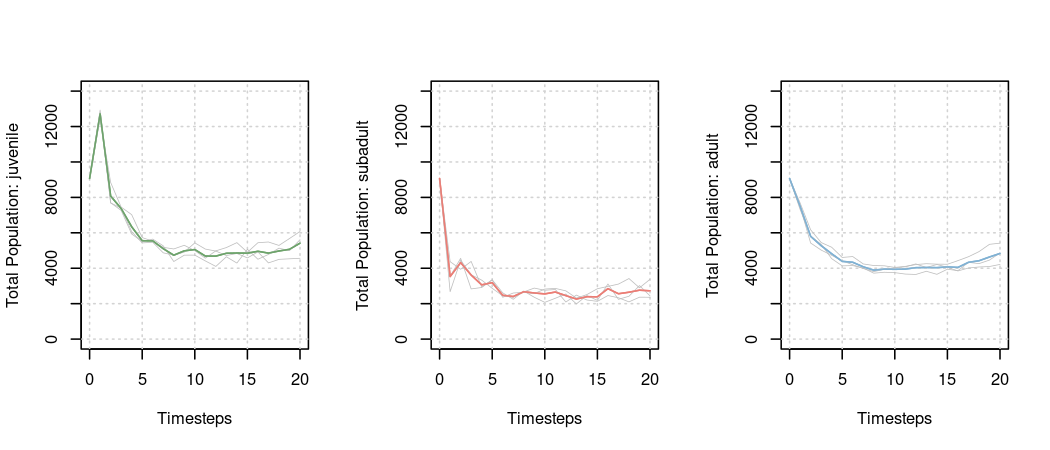A user may also specify a custom function that operates on or defines survival and fecundity in the transition matrix at each timestep. As an example, we have created a custom function below that uses spatial data to define survival and fecundities at each timestep. The function requires the name of the spatial object in the landscape object as an input parameter, and must return a modified transition array (transition matrices for each non-NA cell in the landscape). More information on writing custom functions can be found in a tutorial vignette titled “Creating custom steps functions”. Note, we must also add spatial data (a stack of rasters, “egk_sf” which exists in the steps package) to the landscape object (named “sf_mods”) in order to access it in the simulation. The example stack of rasters “egk_sf” contains 120 layers - twenty timesteps of each of the six non-zero transition values in our matrix - all named with an organisational syntax that the function uses to index. For example, the first layer in the raster stack is named “egk_sf_01_0102”; “01” refers to the first timestep and “0102” refers to the first row and second column position in the transition matrix. Because we are deterministically providing transition values, both global and local environmental stochasticity have been set to zero (defaults).

deterministic_transitions <- function(spatial_object) {

fun <- function (transition_array, landscape, timestep) {

#### This assumes that the spatial layers in the raster stack are named with
#### a particular convention and will not work for all cases.

# get metrics and constructor info
current_timestep <- sprintf("%02i", timestep)
cell_idx <- which(!is.na(raster::getValues(landscape$population[]))) n_cells <- length(which(!is.na(raster::getValues(landscape$population[]))))

# get names of rasters and subset by timestep
names_all <- names(landscape[[spatial_object]])
names_timestep <- grep(paste0("^.*", current_timestep, "\\_"), names_all, value = TRUE)

# populate array:
for (name in names_timestep) {
r <- as.integer(substr(substr(name, nchar(name) - 3, nchar(name)), 1, 2))
c <- as.integer(substr(substr(name, nchar(name) - 1, nchar(name)), 1, 2))
transition_array[r, c, ] <- landscape[[spatial_object]][[name]][cell_idx]
}

#### Return array with required dimensions
transition_array
}

}

egk_landscape <- landscape(population = egk_pop,
suitability = egk_hab,
carrying_capacity = NULL,
"sf_mods" = egk_sf)

egk_pop_dynamics <- population_dynamics(
change = growth(transition_matrix = egk_mat,
transition_function = deterministic_transitions(spatial_object = "sf_mods")),
dispersal = NULL,
modification = NULL,
density_dependence = NULL)

egk_results <- simulation(landscape = egk_landscape,
population_dynamics = egk_pop_dynamics,
habitat_dynamics = NULL,
timesteps = 20,
verbose = FALSE)

plot_pop_trend(egk_results)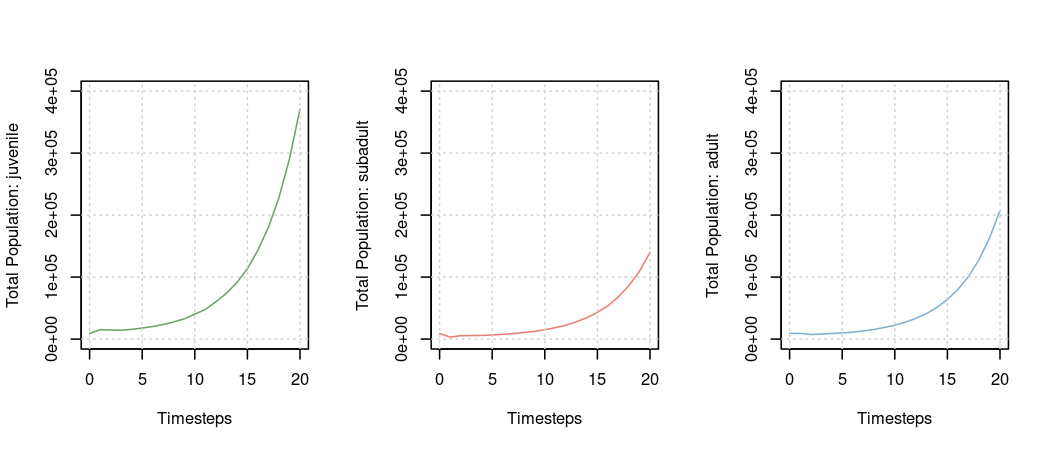Now let’s add some other population dynamics. We can specify ceiling density dependence with the “ceiling_density” function. The population will grow according to the transition matrix until it reaches a ceiling carrying capacity, at which point individuals will die to keep the population at the carrying capacity of the landscape. We must now provide a carrying capacity object in the landscape for this to work - here we provide a spatial layer. By default, all life-stages contribute to density dependence, however, here we specify that it is based on the number of adults only (“stages = 3” in the ceiling_density function). Type ?ceiling_density at the R prompt for more information.

egk_landscape <- landscape(population = egk_pop,
suitability = egk_hab,
carrying_capacity = egk_k)

egk_pop_dynamics <- population_dynamics(change = growth(transition_matrix = egk_mat,
global_stochasticity = egk_mat_stoch),
dispersal = NULL,
modification = NULL,
density_dependence = ceiling_density(stages = 3))

egk_results <- simulation(landscape = egk_landscape,
population_dynamics = egk_pop_dynamics,
habitat_dynamics = NULL,
timesteps = 20,
replicates = 3,
verbose = FALSE)

So far, the populations are only changing within their cells without any movement between cells. We can either specify assisted species movements (i.e. translocations or reintroductions) or natural species movements (dispersal) in the model simulations.

To characterise a translocation, we specify two raster layers that map the locations of where we will take individuals from and where we will place individuals in the landscape. Cell values (integers) specify how many individuals will be added/removed. Origin and destination spatial layers are stored in the landscape object and referenced by name. We use a built-in translocation function to modify the population(s) in a simulation at each specified timestep. The function requires the name of origin and destination layers stored in the landscape object (“origins” and “destinations”), the life-stages to modify, and the timesteps at which to modify the population. In this example, we provide the kangaroo origins (“egk_origins”) and destinations (“egk_destinations”) layers, a life-stage of “3” (only adults moved), and timesteps one, five, ten and fifteen - meaning each translocation will occur every five years in each simulation replicate. Note, if the number of individuals is not available in the landscape when the translocation is applied, a warning will be generated and only the maximum available individuals will be used.

egk_landscape <- landscape(population = egk_pop,
suitability = NULL,
carrying_capacity = NULL,
"origins" = egk_origins,
"destinations" = egk_destinations)

egk_pop_dynamics <- population_dynamics(
change = growth(transition_matrix = egk_mat,
global_stochasticity = egk_mat_stoch),
dispersal = NULL,
modification = translocation(origins_layer = "origins",
destinations_layer = "destinations",
stages = 3,
effect_timesteps = c(1, 5, 10, 15)),
density_dependence = NULL)

egk_results <- simulation(landscape = egk_landscape,
population_dynamics = egk_pop_dynamics,
habitat_dynamics = NULL,
timesteps = 20,
replicates = 3,
verbose = FALSE)

We can also add dispersal to allow kangaroos to move throughout the landscape. There are several built-in dispersal functions and most of them utilise habitat suitability, carrying capacity, or both to determine arrival probabilities - see package help for more information by typing ?population_dispersal_functions at the R prompt. Thus, we will need to provide the appropriate habitat layer in the initial landscape. Below, we use kernel-based dispersal (with a maximum dispersal distance of 5000 meters) and habitat suitability to determine the arrival probabilities:

egk_landscape <- landscape(population = egk_pop,
suitability = egk_hab,
carrying_capacity = egk_k)

egk_pop_dynamics <- population_dynamics(
change = growth(transition_matrix = egk_mat,
global_stochasticity = egk_mat_stoch),
dispersal = kernel_dispersal(
arrival_probability = "suitability",
max_distance = 5000,
dispersal_kernel = exponential_dispersal_kernel(distance_decay = 5000)
),
density_dependence = ceiling_density(stages = 3))

egk_results <- simulation(landscape = egk_landscape,
population_dynamics = egk_pop_dynamics,
habitat_dynamics = NULL,
timesteps = 20,
replicates = 3,
verbose = FALSE)
##  "Kernel-based dispersal utilising available RAM to speed up operations"

Let’s have a look at how the adult population is changing in the first replicate of the simulation:

plot_pop_spatial(egk_results, timesteps = c(1, 10, 20))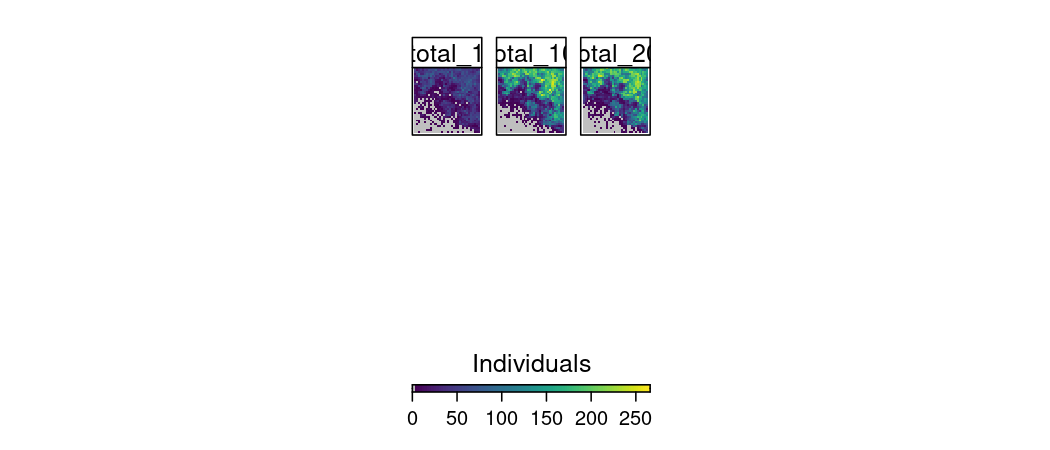The proportion of individuals dispersing can also be specified in the dispersal functions. If dispersal proportions are not specified, the default behaviour is to disperse all individuals in all life-stages. This is done using a default function called all_dispersing(). This default function uses a parameter called “proportions”. If proportions are specified as a single number, then all life-stages disperse with that proportion, however, a vector of proportions (equal in length to the number of life-stages) can also be specified. Note, if a vector of numbers is specified that has fewer elements than life stages, the vector will be recycled to match its number of elements to life stages (i.e. “dispersal_proportion = c(0, 0.5, 1)” with five life-stages will become 0, 0.5, 1, 0, 0.5). Alternatively, a user can provide a custom function to influence the proportions of populations in life-stages dispersing based on features of the landscape. In the example below, the proportions of populations for each life-stage dispersing are based on the carrying capacity - a built-in function of the software. This function uses a global parameter set by “density_dependence” to work out which stages contribute to the carrying capacity of a landscape - below only the adults (stage 3) contribute.

egk_landscape <- landscape(population = egk_pop,
suitability = egk_hab,
carrying_capacity = egk_k)

egk_pop_dynamics <- population_dynamics(
change = growth(transition_matrix = egk_mat,
global_stochasticity = egk_mat_stoch),
dispersal = kernel_dispersal(
arrival_probability = "suitability",
max_distance = 5000,
dispersal_kernel = exponential_dispersal_kernel(distance_decay = 5000),
dispersal_proportion = density_dependence_dispersing()
),
modification = NULL,
density_dependence = ceiling_density(stages = 3))

egk_results <- simulation(landscape = egk_landscape,
population_dynamics = egk_pop_dynamics,
habitat_dynamics = NULL,
timesteps = 20,
replicates = 3,
verbose = FALSE)

For kernel-based dispersal, specifying a single number for the “max_distance” parameter will indicate maximum dispersal distances for all life-stages. The ‘number of cells’ representation of distance should be carefully considered when specifying custom distance kernel functions. For example, below we have specified an exponential distance decay function where “r” represents spatial units - in this case 5000 meters (10 cells). How we specify the function will depend on the ecology of the species and known dispersal distances. The function should return a value close to zero when the maximum dispersal distances is specified for “r”:

egk_landscape <- landscape(population = egk_pop,
suitability = egk_hab,
carrying_capacity = egk_k)

egk_pop_dynamics <- population_dynamics(
change = growth(transition_matrix = egk_mat,
global_stochasticity = egk_mat_stoch),
dispersal = kernel_dispersal(arrival_probability = "suitability",
max_distance = 5000,
dispersal_kernel = function (r) exp(-r / 2000),
dispersal_proportion = density_dependence_dispersing()),
modification = NULL,
density_dependence = ceiling_density(stages = 3))

egk_results <- simulation(landscape = egk_landscape,
population_dynamics = egk_pop_dynamics,
habitat_dynamics = NULL,
timesteps = 20,
replicates = 3,
verbose = FALSE)

For individual-based movements, we also provide a cellular automata-based dispersal function. Because this is an individual-based model, a dispersal kernel is not required. Specifying a single number for the “max_cells” parameter will indicate the maximum number of cells movements (traversed around the landscape), for each dispersing individual, for all life-stages. A vector of max_cells (equal in length to the number of life-stages) can also be specified. In the example below, no juveniles (stage 1) disperse, subadults (stage 2) only disperse up to 10 cells (5000 meters), and adults (stage 3) disperse up to 20 cells (10000 meters):

egk_landscape <- landscape(population = egk_pop,
suitability = egk_hab,
carrying_capacity = egk_k)

egk_pop_dynamics <- population_dynamics(
change = growth(transition_matrix = egk_mat,
global_stochasticity = egk_mat_stoch),
dispersal = cellular_automata_dispersal(
max_cells = c(0, 10, 20)
),
modification = NULL,
density_dependence = ceiling_density(stages = 3))

egk_results <- simulation(landscape = egk_landscape,
population_dynamics = egk_pop_dynamics,
habitat_dynamics = NULL,
timesteps = 20,
replicates = 3,
verbose = FALSE)

Thus far, the kangaroo population is the only thing to be dynamically changing in the landscape, however, often habitat suitability also changes due to disturbances. To characterise habitat disturbance, we can use a series of raster layers that map the locations and severity of disturbances. Each disturbance raster will be multiplied with the original habitat suitability layer and thus contain values that represent appropriate ranges - zero representing the maximum disturbance and one representing no disturbance. Note, the number of disturbance layers must match the intended number of timesteps in a single simulation. There is an existing spatial dataset of fires in the steps package (“egk_fire”) which we store in the landscape object. We use a pre-defined fire_effects function to modify the habitat suitability in the simulation at each timestep. The function requires the name of fire layers stored in the landscape object, and an effect time which specifies the number of timesteps that each disturbance layer acts on the habitat suitability (in diminishing intensity). The effect of fires can be cumulative, so if there are two fires in the same cell in succession (i.e. in a shorter space of time than the effect time) then the habitat suitability will equal suitability multiplied by both the first and second disturbance effects. In this example, we provide the kangaroo fire disturbance input name (“fires”), and an effect time of five - meaning each fire layer will affect the habitat suitability (in linear decreasing intensity based on the regeneration function) for five timesteps in each simulation replicate. All functions that act on the habitat must be passed in as a list in the simulation call in the order to be executed:

egk_landscape <- landscape(population = egk_pop,
suitability = egk_hab,
carrying_capacity = egk_k,
"fires" = egk_fire)

egk_pop_dynamics <- population_dynamics(
change = growth(transition_matrix = egk_mat,
global_stochasticity = egk_mat_stoch),
dispersal = kernel_dispersal(
arrival_probability = "suitability",
max_distance = 5000,
dispersal_kernel = exponential_dispersal_kernel(distance_decay = 5000)
),
modification = NULL,
density_dependence = ceiling_density(stages = 3))

egk_results <- simulation(landscape = egk_landscape,
population_dynamics = egk_pop_dynamics,
habitat_dynamics = list(
fire_effects(fire_layers = "fires",
effect_time = 5,
regeneration_function = function (time) {-time})
),
timesteps = 20,
replicates = 3,
verbose = FALSE)

plot_pop_trend(egk_results)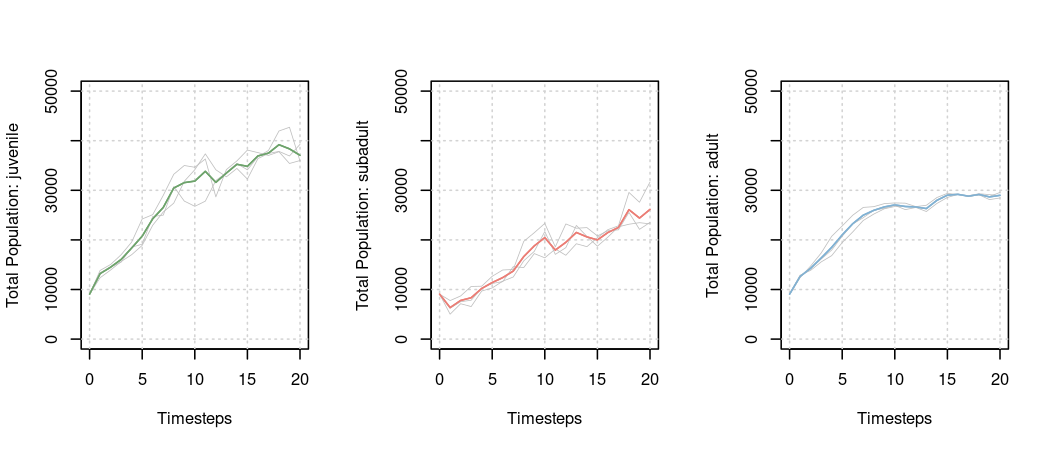And now let’s have a look at how the adult population is changing with fires occurring in the landscape:

plot_pop_spatial(egk_results, stage = 3, timesteps = c(1, 10, 20))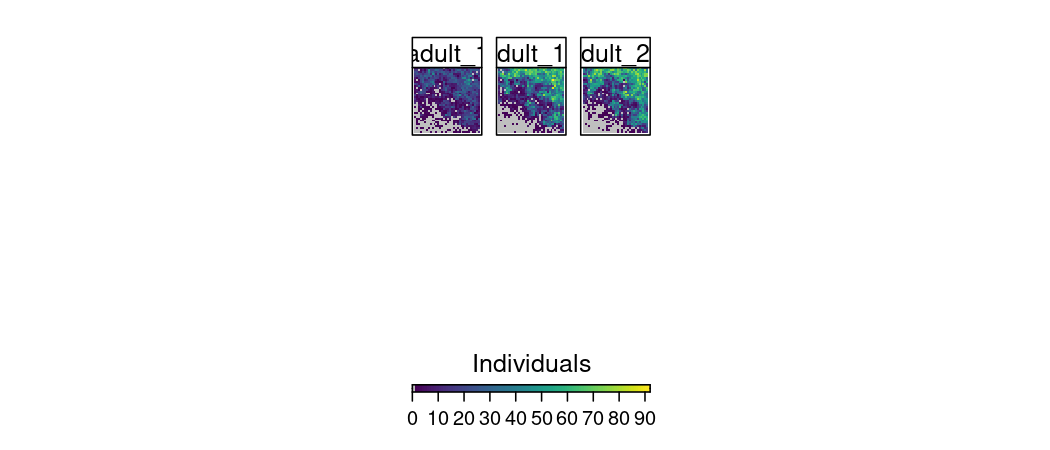Since we are using habitat dynamics we may want to have a look at how the habitat suitability changes in a landscape; it is possible to view the habitat suitability throughout a single simulation:

plot_hab_spatial(egk_results, timesteps = c(1, 10, 20))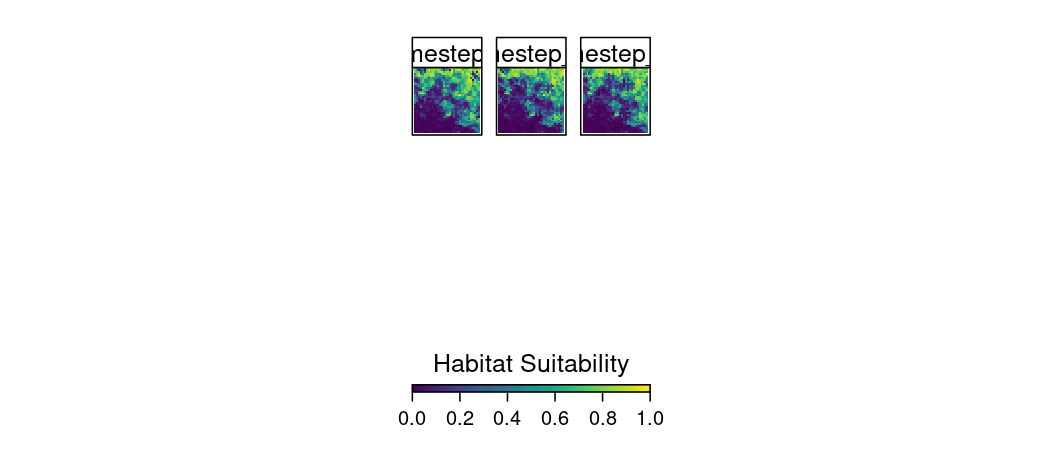In all of the examples above, carrying capacity has remained unchanged throughout a simulation. However, carrying capacity is often derived from dynamically changing habitat suitabilities. A function of this relationship can be specified in place of the carrying_capacity raster layer when constructing the landscape object. Internally, the carrying capacity raster will be constructed by applying the function to the habitat suitability raster at each timestep. Here we have created a function that produces descending integers from our hypothetical maximum carrying capacity population of 75 based on decreasing values of habitat suitability (one to zero):

carrying_cap_fun <- function (landscape, timestep) {

fun <- function(suitability) {
75 - round(75 * dlogis(suitability, scale = 0.25))
}

suit <- landscape\$suitability
if (raster::nlayers(suit) > 1) {
suit <- suit[[timestep]]
}

raster::calc(suit, fun)

}

suit_seq <- seq(0, 1, 0.1)
plot(suit_seq, 75 - round(75 * dlogis(suit_seq, scale = 0.25)), type = 'l')

egk_landscape <- landscape(population = egk_pop,
suitability = egk_hab,
carrying_capacity = carrying_cap_fun,
fires = egk_fire)

egk_pop_dynamics <- population_dynamics(
change = growth(transition_matrix = egk_mat,
global_stochasticity = egk_mat_stoch),
dispersal = kernel_dispersal(
arrival_probability = "suitability",
max_distance = 5000,
dispersal_kernel = exponential_dispersal_kernel(distance_decay = 5000)
),
modification = NULL,
density_dependence = ceiling_density(stages = 3))

egk_results <- simulation(landscape = egk_landscape,
population_dynamics = egk_pop_dynamics,
habitat_dynamics = list(fire_effects(fire_layers = "fires",
effect_time = 5,
regeneration_function =
function (time) {-time})),
timesteps = 20,
replicates = 3,
verbose = FALSE)

One may specify multiple disturbances affecting the habitat throughout a simulation. To do so, simply add habitat dynamic functions to the list in the simulation function - note, these will be executed in the order that they are listed. To demonstrate this functionality, we have added another disturbance - a road being constructed through the landscape over five years. There is an existing spatial dataset of a road in the steps package (“egk_road”) which we store in the landscape object. Since we have already accommodated the movement of the road construction across the landscape, and the vegetation will not regenerate, we specify the effect time as one:

egk_landscape <- landscape(population = egk_pop,
suitability = egk_hab,
carrying_capacity = carrying_cap_fun,
"fires" = egk_fire,

egk_pop_dynamics <- population_dynamics(
change = growth(transition_matrix = egk_mat,
global_stochasticity = egk_mat_stoch),
dispersal = kernel_dispersal(
arrival_probability = "suitability",
max_distance = 5000,
dispersal_kernel = exponential_dispersal_kernel(distance_decay = 5000)
),
modification = NULL,
density_dependence = ceiling_density(stages = 3))

egk_results <- simulation(landscape = egk_landscape,
population_dynamics = egk_pop_dynamics,
habitat_dynamics = list(
fire_effects(fire_layers = "fires",
effect_time = 5,
regeneration_function = function (time) {-time}),
effect_time = 1)
),
timesteps = 20,
replicates = 3,
verbose = FALSE)

The simulation results object (egk_results in our examples) is a list of lists containing spatial objects and is depicted by the following tree diagram:

• Replicate (a)
• Timestep (b)
• Population Raster Stack (c)
• Life-Stage Raster (d)
• Habitat Suitability Raster (or Stack) (c)
• Habitat Raster (if stack is used) (d)
• Carrying Capacity Raster (c)
• Other Raster Stack (c)
• Raster (d)

To access the different components from the results object the following syntax is used:

result_object[[a]][[b]][[c]][[d]]

where a through d represent numbers denoting replicate, timestep, or object indices. All values will equal one or a larger positive integer depending on the simulation setup. The landscape object (denoted by c) will always be structured in the same order where position one is the population, position two is the habitat suitability, and position three is the carrying capacity. Positions beyond these are reserved for additional spatial data added to the initial landscape object.

For example, to return the population raster for the first life-stage in timestep five of the second replicate the following syntax would be used:

egk_results[][][][]
## class      : RasterLayer
## dimensions : 35, 36, 1260  (nrow, ncol, ncell)
## resolution : 500, 500  (x, y)
## extent     : 319000, 337000, 5817000, 5834500  (xmin, xmax, ymin, ymax)
## crs        : +proj=utm +zone=55 +south +ellps=GRS80 +towgs84=0,0,0,0,0,0,0 +units=m +no_defs
## source     : memory
## names      : juvenile
## values     : 0, 90  (min, max)

To return the habitat suitability for the third timestep of the second replicate (note d is not used in this case):

egk_results[][][]
## class      : RasterLayer
## dimensions : 35, 36, 1260  (nrow, ncol, ncell)
## resolution : 500, 500  (x, y)
## extent     : 319000, 337000, 5817000, 5834500  (xmin, xmax, ymin, ymax)
## crs        : +proj=utm +zone=55 +south +ellps=GRS80 +towgs84=0,0,0,0,0,0,0 +units=m +no_defs
## source     : memory
## names      : layer
## values     : 0.001544298, 0.9652193  (min, max)

Alternatively, the names of the raster objects can be used:

egk_results[][][["suitability"]]
## class      : RasterLayer
## dimensions : 35, 36, 1260  (nrow, ncol, ncell)
## resolution : 500, 500  (x, y)
## extent     : 319000, 337000, 5817000, 5834500  (xmin, xmax, ymin, ymax)
## crs        : +proj=utm +zone=55 +south +ellps=GRS80 +towgs84=0,0,0,0,0,0,0 +units=m +no_defs
## source     : memory
## names      : layer
## values     : 0.001544298, 0.9652193  (min, max)

Based on this structure, we have also provided an extraction function to return components of a simulation results object. For example, to return the same object as in the previous example:

extract_spatial(egk_results, replicate = 2, timestep = 3, landscape_object = "suitability")
## class      : RasterLayer
## dimensions : 35, 36, 1260  (nrow, ncol, ncell)
## resolution : 500, 500  (x, y)
## extent     : 319000, 337000, 5817000, 5834500  (xmin, xmax, ymin, ymax)
## crs        : +proj=utm +zone=55 +south +ellps=GRS80 +towgs84=0,0,0,0,0,0,0 +units=m +no_defs
## source     : memory
## names      : layer
## values     : 0.001544298, 0.9652193  (min, max)

By default, the extract function will return a population raster (‘landscape object = 1’ or ‘landscape object = “population”’), for the first life-stage (“stage = 1”), for the first iteration (“timestep = 1”), for the first simulation run (“replicate = 1”). These values can be specified accordingly to return the spatial object of your choosing. Here, we plot the default object from the extract function:

par(mar = c(0.1, 0.1, 0.1, 0.1))
plot(extract_spatial(egk_results), box = FALSE, axes = FALSE, col = viridis(100))